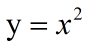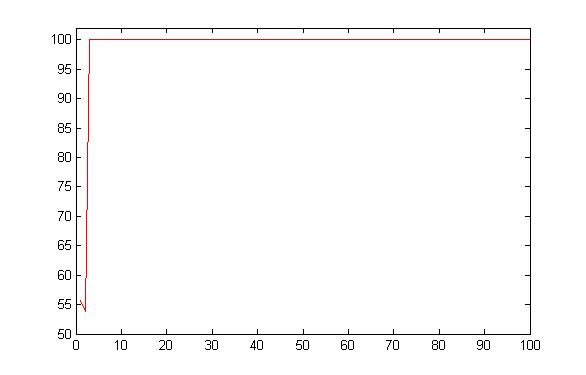• 用于遗传算法了解的一些函数例子,没有代码，只是给出一些函数练手
• 目录概述编码初始群体的产生适应度计算选择运算交叉运算变异运算测试效果参考资料一，概述本文在理解遗传算法的基本原理的基础上，使用Java语言写了一个小程序来简单实现用遗传算法求解二次函数f(x,y)=xx+yy (x和y的...
我的《自然计算》作业，搬上来。目录概述编码初始群体的产生适应度计算选择运算交叉运算变异运算测试效果参考资料一，概述本文在理解遗传算法的基本原理的基础上，使用Java语言写了一个小程序来简单实现用遗传算法求解二次函数f(x,y)=xx+yy (x和y的取值范围为1到127的正整数)的最大值。完整源码见已上传至我的github，详情请点击此链接：查看本项目全部代码二，编码重述一下本文求解的问题：image.png由于遗传算法的运算对象是表示个体的符号串，所以必须把变量x,y编码为一种符号串。在这里采用无符号二进制整数来表示。因x,y的取值范围为1到127,，则可用两个7位二进制数来分别表示它们。而一个基因就是一个14位二进制串。例如，基因型00000001111111所对应的表现型是：x=0,y=127。在代码中的具体体现为：设计类Chromosome，其具有私有整形字段x,y表示x,y的十进制值、String字段gene表示二进制串。并使用常量定义其最大值或最大长度。代码如下：public static final int GENG_LENGTH = 14;public static final int MAX_X = 127;public static final int MAX_Y = 127;private int x,y;private String gene;三，初始群体的产生遗传算法是对群体进行的进化操作，所以第一步要产生一个初始种群，再进行后续的交叉、变异、选择等操作。在具体实现上，初始种群的产生较为简单。首先对类Chromosome进行构造函数的编写，主要写了以下两种构造函数：public Chromosome(String gene)   //给定基因串构造染色体public Chromosome(int x,int y)    //给定表现型构造染色体在这里用到了第二个构造函数，首先随机产生两个1到127之间的数作为X,Y，再调用构造函数生成新染色体并添加到初始种群中去。如此重复，直到种群规模达到要求。具体代码如下:public static ArrayList initGroup(int size) {ArrayList list = new ArrayList();Random random = new Random();for(int i = 0; i < size; i++) {int x = random.nextInt() % 128;int y = random.nextInt() % 128;x = x < 0? (-x):x;y = y < 0? (-y):y;list.add(new Chromosome(x,y));}return list;}四，适应度计算遗传算法中以个体适应度评价其优劣程度，其最终目的是选择出适应度较高的个体。本文所求解问题，目标函数总取非负值，并且是以求函数最大值为优化目标，故可直接利用目标函数值作为个体的适应度。具体求解代码如下：public int calcFitness() {return x*x+y*y;}五，选择运算选择运算把当前的群体中适应度较高的个体按照某种规则或模型遗传到下一代个体中。这里我们使用课堂上讲的“轮盘赌”的方式进行选择。“轮盘赌”的思想这里不再赘述，只说一下JAVA代码的具体实现过程。首先计算种群中每个个体的适应度保存到一个数组里。在这基础上计算“累加适应度”，即第一个适应度为原第一个适应度，第二个为前两个的和，第三个为前三个的和，以此类推。再将此数组每个元素除以总的适应度。然后进行选择，选择次数和设定好的种群规模相同(这样便可以控制种群的规模不会无限的上涨)。每次选择时，先产生一个0到1之间的随机数。在上述数组中遍历找到第一个大于此随机数的元素，这个元素对应的个体被选择。具体代码如下：public static ArrayList selector(ArrayList fatherGroup,int sonGroupSize){ArrayList sonGroup = new ArrayList();int totalFitness = 0;double[] fitness = new double[fatherGroup.size()];for(Chromosome chrom : fatherGroup) {totalFitness += chrom.calcFitness();}int index = 0;//计算适应度for(Chromosome chrom : fatherGroup) {fitness[index] = chrom.calcFitness() / ((double)totalFitness);index++;}//计算累加适应度for(int i = 1; i < fitness.length; i++) {fitness[i] = fitness[i-1]+fitness[i];}//轮盘赌选择for(int i = 0; i < sonGroupSize; i++) {Random random = new Random();double probability = random.nextDouble();int choose;for(choose = 1; choose < fitness.length - 1; choose++) {if(probability < fitness[choose])break;}sonGroup.add(new Chromosome(fatherGroup.get(choose).getGene()));}return sonGroup;}六，交叉运算交叉运算是遗传算法中产生新个体的主要操作过程，它以某一概率相互交换某两个个体之间的部分染色体。本文采用单点交叉的方法，具体操作过程是：先对群体进行随机配对；其次随机设置交叉点位置；最后再相互交换配对染色体之间的部分基因。具体的算法流程按照老师课件上讲的，这里也不赘述了。只说一下具体代码实现原理。这个算法的要点在于随机配对个体进行交叉、字符串的拼接操作和生成新个体。随机配对可以使用一个do while循环，选择两个不同的个体。然后随机选取交叉点，进行两个基因字符串的交叉。最后生成新的个体，采用以下构造函数生成新的个体：public Chromosome(String gene)。具体代码如下：public static ArrayList corssover(ArrayList fatherGroup,double probability) {ArrayList sonGroup = new ArrayList();sonGroup.addAll(fatherGroup);Random random = new Random();for(int k = 0; k < fatherGroup.size() / 2; k++) {if(probability > random.nextDouble()) {int i = 0,j = 0;do {i = random.nextInt(fatherGroup.size());j = random.nextInt(fatherGroup.size());} while(i == j);int position = random.nextInt(Chromosome.GENG_LENGTH);String parent1 = fatherGroup.get(i).getGene();String parent2 = fatherGroup.get(j).getGene();String son1 = parent1.substring(0, position) +                                          parent2.substring(position);String son2 = parent2.substring(0, position) +                                          parent1.substring(position);sonGroup.add(new Chromosome(son1));sonGroup.add(new Chromosome(son2));}}return sonGroup;}七，变异运算变异运算是对个体的某一个或某一些基因按某一较小的概率进行改变，它也是产生新个体的一种操作方法。本文中我们采用如下方式进行变异：遍历群体中所有个体的所有基因位，以一个较小的概率对每个基因位进行变异操作即1变成0,0变成1。在Java代码中具体实现时，我们先编写一个函数对一个个体的基因进行改变，即用新基因串代替旧基因串。该函数编写如下：public void selfMutation(String newGene) {if(newGene.length() != Chromosome.GENG_LENGTH)return;this.gene = newGene;String xStr = newGene.substring(0, Chromosome.GENG_LENGTH/2);String yStr = newGene.substring(Chromosome.GENG_LENGTH/2);this.x = Integer.parseInt(xStr,2);this.y = Integer.parseInt(yStr,2);}然后，按照上述说法对每一位基因进行遍历，按指定概率进行变异操作。具体代码如下所示：public static void mutation(ArrayList fatherGroup,double probability) {Random random = new Random();Chromosome bestOne = Chromosome.best(fatherGroup);fatherGroup.add(new Chromosome(bestOne.getGene()));for(Chromosome c : fatherGroup) {String newGene = c.getGene();for(int i = 0; i < newGene.length();i++){if(probability > random.nextDouble()) {String newChar = newGene.charAt(i) == '0'?"1":"0";newGene = newGene.substring(0, i) + newChar +                                   newGene.substring(i+1);}}c.selfMutation(newGene);}}八，测试效果以上实现了所有子模块。下面使用上述模块进行实际的遗传算法求解操作。首先产生初始种群，按照交叉、变异、选择的顺序进行循环，直到选出符合要求的个体，这里设置的条件为适应度大于等于32258时终止，这也是本题中能达到的最大适应度。final int GROUP_SIZE = 20;//种群规模final double CORSSOVER_P = 0.6;//交叉概率final double MUTATION_P = 0.01;//变异概率ArrayList group =                                               Chromosome.initGroup(GROUP_SIZE);Chromosome theBest;do{group = Chromosome.corssover(group, CORSSOVER_P);Chromosome.mutation(group, MUTATION_P);group = Chromosome.selector(group, GROUP_SIZE);theBest = Chromosome.best(group);System.out.println(theBest.calcFitness());}while(theBest.calcFitness() < 32258);如上述代码所示，在每次迭代后输出当前种群中的最优个体。下面进行了三次测试。最优个体适应度随种群代数变化图，如下图所示(三次的结果)。可以看出随着迭代次数的增加，种群中的最优个体适应度总体呈上升趋势，最终一般在50代之内就会找到满足要求的个体。image.pngimage.pngimage.png参考资料：
展开全文• 8个测试例子来自论文： 基于学习的遗传算法及其在布局中的应用.pdf 著名的 De Jong function, schaffer function, six-hump camel back function, Shubert function, 算法效果非常好！ （自己写的代码）
• 标准的量子遗传算法容易陷入局部最优，且在定义域范围较广的函数寻优中易出现优化精度不高的情况。将量子交叉与模拟退火操作适当地加入量子遗传算法中可以有效地避免算法陷入局部最优解。根据第一次的优化结果，缩小...
• 遗传算法（Genetic Algorithm, GA）是模拟达尔文生物进化论的自然选择和遗传学机理的生物进化过程的计算模型，是一种通过模拟自然进化过程搜索最优解的方法。 简而言之，遗传算法就是通过每次选择比较好的个体进入...
遗传算法（Genetic Algorithm, GA）是模拟达尔文生物进化论的自然选择和遗传学机理的生物进化过程的计算模型，是一种通过模拟自然进化过程搜索最优解的方法。
简而言之，遗传算法就是通过每次选择比较好的个体进入下一次循环来保证每一轮解的最优特性，其核心思想有以下几个方面。
（1）初始化值（染色体），转化成二进制的形式（为了方便交叉和变异）
（2）使用轮盘赌算法设计策略来选择下一轮进入循环的染色体（只要是为了防止当前适应度值不太好的染色体直接被淘汰）
（3）复制染色体（保证每一轮染色体的条数和初始染色体条数一样，比如初始染色体有4条，通过轮盘赌算法后只选择了3条，那么剩下的一条一般是复制当前轮中比较好的染色体，当然，这个时候4条染色体中有2条是一样的，所以要进行交叉和变异）
（4）交叉，交叉的策略很多，可以选择任意两条染色体相交叉（其实就是交换染色体对应的二进制的某些值，比如选择第一条染色体对应的二进制前3个值和第二条染色体对应的二进制前3个值对换，交叉的目的在于检验这些比较好的染色体相互组合后是否能达到求解要求，也可以说是保留最优染色体基因，其实交叉后也有可能会变差）
（5）变异，当染色体相互交叉后并不能找到最优值，就需要变异，变异就是改变某条染色体中对应的基因值，把染色体对应的二进制中某个1变成0，或者0变成1，变异策略也有很多，需要几个基因变异因问题不同而不同。
交叉和变异都是为了防止进入局部最优。
为了方便说明，根据遗传算法原理，求解二次函数极大值问题,定义域为（1,10）步骤为：
（1）根据定义域，初始化染色体数目和值
（2）计算每条染色体对应的y值
（3）根据轮盘赌算法选择下一次要遗传的染色体，染色体数目不够就复制
（4）二进制编码染色体
（5）交叉
（6）变异，更新下一轮循环染色体
（7）没有达到最大循环次数，返回（2），否则，输入目标值。
另外，交叉和变异后最好都检验下新的染色体是否还在定义域范围，如果超出范围，要调整。
代码如下：
clc;
clear all;
%遗传算法求y=x1^2在区间[1 10]的最大值，求y的值和x的值
%初始种群规模M=4
%终止迭代次数
%交叉概率
%变异概率
% 复制 、交叉、变异
beginNum=1+(10-1)*rand(1,4)%随机生成4个初始值，染色体
%计算初始使用度值
T=100;%最大循环次数
tt=1;
maxsyd=[];
while tt<=T
beginNum
zydz=[];
for j=1:length(beginNum)
zydz(j)=beginNum(j)^2;
end

%      if max(zydz)==100
%          beginNum;
%          break;
%      end

%根据轮盘赌算法决定下次遗传的
%计算适应度的选择概率
p=[];
for z=1:length(zydz)
p(z)=zydz(z)/sum(zydz);
end
p;
%计算累加概率q
q=[];
q(1)=p(1);
for y=2:length(p)
q(y)=p(y)+ q(y-1);
end
q;
gxnum=[];
r=rand(1,length(beginNum));%在[0 1]之间产生随机数字r产生length(beginNum)个
for k=1:length(r)
if r(k)<=q(1)
gxnum=[gxnum beginNum(1)];
end
for kk=2:length(q) %每个随机数字r在q中的范围
if q(kk-1)<r(k)&&r(k)<=q(kk)
gxnum=[gxnum beginNum(kk)];
end
end
end
gxnum=unique(gxnum,'stable');
%判断是否需要复制
if length(gxnum)<length(beginNum)
% 选择复制优势染色体
while length(gxnum)~=length(beginNum)
gxnum=[gxnum max(beginNum)];
end
end

m=8;
newbeginenum={};%转换成二进制后的初始数，方便变异和交叉操作
for i=1:length(gxnum)
n=beginNum(i);
a=dec2bin(n*2^m);
%     b=[a(1:end-m),'.',a(end-m+1:end)];
% str2double(a)
newbeginenum{i}=a;
end
t=char(newbeginenum(1));%转成字符串类型
%所有二进制的位数必须一样，不足的在前面补0
yycount=0;
for yy=1:length(newbeginenum)
% 找最长位数的二进制
yyt1=length(char(newbeginenum(yy)));
if yyt1>yycount
yycount=yyt1;
end
end
for pj=1:length(newbeginenum)
yy2=length(char(newbeginenum(pj)));
if yy2<yycount
tempj(1:yycount-yy2)='0';
newbeginenum{pj}=strcat(tempj,char(newbeginenum(pj)));%更新newbeginenum
end
end

nm=2;%交换前2位
jcnewbeginenum={};%交叉产生新的染色体jcnewbeginenum
for nk=1:2:length(newbeginenum)-1
t1=char(newbeginenum(nk));
t2=char(newbeginenum(nk+1));
tz1=t1(1:nm);
tz2=t2(1:nm);
% 交换
tztemp=tz1;
t1(1:nm)=tz2;
t2(1:nm)=tztemp;
jcnewbeginenum{nk}=t1;
jcnewbeginenum{nk+1}=t2;
end
%判断变异是否超出定义域范围

jcnewbeginenum(3);%
% 变异，根据要求，设置变异概率10%，
%变异基因个数jycount
byhbegine={}; %变异后的值，等下记得判断是否超出定义域的范围
for jyk=1:length(jcnewbeginenum)
jycount=ceil(length(char(jcnewbeginenum(jyk)))*0.1);
% rk=randperm(length(char(jcnewbeginenumjyk))),jycount));%产生变异基因的位置
rk=randperm(length(char(jcnewbeginenum(jyk))),jycount);
% 替换变异位置
bywz=char(jcnewbeginenum(jyk));
for byw=1:length(rk)
if strcmp(bywz(rk(byw)),'0')
bywz(rk(byw))='1';
else
bywz(rk(byw))='1';

end
%     bywz(rk(byw))=~bywz(rk(byw));
end
byhbegine{jyk}=bywz;
end
%把变异后的值转换成十进制替换初始值
zzbyhbegine=[];
for ncs=1:length(byhbegine)
tempaa=char(byhbegine(ncs));
%二进制转换成十进制
zzbyhbegine(ncs)=bin2dec(tempaa(1:end-m))+bin2dec(tempaa(end-m+1:end))/2^m;
end
beginNum=zzbyhbegine;
for bj=1:length(beginNum)
if beginNum(bj)<1
beginNum(bj)=1;
end
if beginNum(bj)>10
beginNum(bj)=10;
end
end
tt=tt+1;
maxsyd=[maxsyd max(zydz)];
end
xsyd=1:100;
plot(xsyd,maxsyd,'r-')

运行结果可以看到，在定义域为（1,10）中，遗传算法收敛速度还是非常快的（大约在第三轮就已经收敛了）。另外，在实验过程中，由于变异和交叉策略选择的原因，发现该算法还是会陷入局部最优，其陷入局部最优的概率为约为5%。


展开全文matlab
• 在使用基本遗传算法的基础上做一些改进，可以在代码里修改是否选择改进方式以及选择常用测试函数的索引来更好地求得函数的最优值。
• 这个测试函数，可以用来测试遗传算法，粒子群算法、模拟退火算法等智能算法
• 简单的遗传算法函数最大值，VS2005-c#工程源代码，欢迎测试和修改，传播请保留个人信息，期待和大家多多交流！
• 网上看到了一个比较不错的讲解遗传算法的帖子，链接如下http://blog.csdn.net/b2b160/article/details/4680853但是却没有贴源代码，正好最近闲来无事，就尝试写了下代码实现，测试了几次，寻优结果都能达到了二元...
网上看到了一个比较不错的讲解遗传算法的帖子，链接如下http://blog.csdn.net/b2b160/article/details/4680853但是却没有贴源代码，正好最近闲来无事，就尝试写了下代码实现，测试了几次，寻优结果都能达到了二元函数最大值98，如下所示#include #include #include #include #include #include #include #include using namespace std;#define MAXLENGTH 1000#define DNANUMBER 30#define DNALENGTH 6#define ITERATIONNUMBER 100int generic[DNANUMBER][MAXLENGTH];int select[DNANUMBER][MAXLENGTH];int FitNess[DNANUMBER];float FitNessProportion[DNANUMBER];//二次函数为f(x1,x2)=x1^2+x2^2；遗传数组为待求解的串组合int fitMax;void InitDna(){//对所有的染色体进行随机初始化int i,j;fitMax=INT_MIN;srand(time(NULL));for(i=0;i{for(j=0;j{if(rand()%2==0){generic[i][j]=1;}elsegeneric[i][j]=0;}}}int BinaryToDecimal(int index,int start,int end){int ret=0;int i;int mul=1;for(i=end;i>=start;i--){if(generic[index][i]==1){ret+=mul;}mul=mul*2;}return ret;}int Decode(int index){int i=0;int x1,x2;x1=BinaryToDecimal(index,0,DNALENGTH/2-1);x2=BinaryToDecimal(index,DNALENGTH/2,DNALENGTH-1);return x1*x1+x2*x2;}void CopyGenericToSelect(int srcIndex,int dstIndex){int i=0;for(i=0;i{select[dstIndex][i]=generic[srcIndex][i];}}void CopySelecToGeneric(int srcIndex,int dstIndex){int i=0;for(i=0;i{generic[dstIndex][i]=select[srcIndex][i];}}void  SelectDna(){//进行适应度计算 然后填充FitNess和FitNessProportion数组int i,j;int fitSum=0;for(i=0;i{FitNess[i]=Decode(i);fitMax=std::max(fitMax,FitNess[i]);fitSum+=FitNess[i];}for(i=0;i{FitNessProportion[i]=0;FitNessProportion[i]+=FitNess[i];}//生成和DNA数目相同的随机数，查看其落在FitNessProprotion哪个区间，并选择其对应的区域，这就是经典的轮盘赌选择方法srand(time(NULL));int mask[DNANUMBER];for(i=0;i{mask[i]=0;}float randNumber;for(i=0;i{randNumber=rand()/RAND_MAX;for(j=0;j{if(randNumber{mask[j]++;break;}}}//根据mask数组情况来进行染色体复制  比如mask数组为 1 1 2 0 1 ，就把1，2，3，5号染色体复制回原染色体组，其中3号染色体复制两次int count=0;for(i=0;i{while(mask[i]!=0){CopyGenericToSelect(mask[i],count);count++;mask[i]--;}}//将选择后的select矩阵写回到原generic矩阵for(i=0;i{CopySelecToGeneric(i,i);}}void Swap(int index){int i,j;int swapIndex;int temp;srand(time(NULL));swapIndex=rand()%DNANUMBER;for(i=0;i{temp=generic[index][i];generic[index][i]=generic[swapIndex][i];generic[swapIndex][i]=temp;}}void CrossDna(){//该函数为交叉操作//对dna矩阵进行随机分组int i,j;for(i=0;i{Swap(i);}//两两一组,进行交叉srand(time(NULL));int crossPoint;int temp;for(i=0;i{crossPoint=rand()%DNALENGTH;for(j=crossPoint;j{temp=generic[i][j];generic[i][j]=generic[i+1][j];generic[i+1][j]=temp;}}}void VariateDna(){srand(time(NULL));int variaPoint=rand()%DNALENGTH;int i;for(i=0;i{if(generic[i][variaPoint]==1)generic[i][variaPoint]=0;elsegeneric[i][variaPoint]=1;}}void Generic(){int i,j,k;InitDna();for(i=0;i{SelectDna();CrossDna();VariateDna();}cout<}int main(){int i;for(i=0;i<50;i++){Generic();}return 0;}
展开全文• 一，概述本文在理解遗传算法的基本原理的基础上，使用Java语言写了一个小程序来简单实现用遗传算法求解二次函数f(x,y)=x*x+y*y (x和y的取值范围为1到127的正整数)的最大值。完整源码见已上传至我的github，详情请...
我的《自然计算》作业，搬上来。
（原来发布到简书了，现在搬过来）

目录

概述
编码
初始群体的产生
适应度计算
选择运算
交叉运算
变异运算
测试效果
参考资料
一，概述

本文在理解遗传算法的基本原理的基础上，使用Java语言写了一个小程序来简单实现用遗传算法求解二次函数f(x,y)=x*x+y*y (x和y的取值范围为1到127的正整数)的最大值。完整源码见已上传至我的github，详情请点击此链接：查看本项目全部代码

二，编码

重述一下本文求解的问题：由于遗传算法的运算对象是表示个体的符号串，所以必须把变量x,y编码为一种符号串。在这里采用无符号二进制整数来表示。因x,y的取值范围为1到127,，则可用两个7位二进制数来分别表示它们。而一个基因就是一个14位二进制串。例如，基因型00000001111111所对应的表现型是：x=0,y=127。在代码中的具体体现为：设计类Chromosome，其具有私有整形字段x,y表示x,y的十进制值、String字段gene表示二进制串。并使用常量定义其最大值或最大长度。代码如下：

public static final int GENG_LENGTH = 14;
public static final int MAX_X = 127;
public static final int MAX_Y = 127;
private int x,y;
private String gene;

三，初始群体的产生

遗传算法是对群体进行的进化操作，所以第一步要产生一个初始种群，再进行后续的交叉、变异、选择等操作。在具体实现上，初始种群的产生较为简单。首先对类Chromosome进行构造函数的编写，主要写了以下两种构造函数：
public Chromosome(String gene)   //给定基因串构造染色体
public Chromosome(int x,int y)    //给定表现型构造染色体
在这里用到了第二个构造函数，首先随机产生两个1到127之间的数作为X,Y，再调用构造函数生成新染色体并添加到初始种群中去。如此重复，直到种群规模达到要求。具体代码如下:

    public static ArrayList<Chromosome> initGroup(int size) {
ArrayList<Chromosome> list = new ArrayList<Chromosome>();
Random random = new Random();
for(int i = 0; i < size; i++) {
int x = random.nextInt() % 128;
int y = random.nextInt() % 128;
x = x < 0? (-x):x;
y = y < 0? (-y):y;
list.add(new Chromosome(x,y));
}
return list;
}

四，适应度计算

遗传算法中以个体适应度评价其优劣程度，其最终目的是选择出适应度较高的个体。本文所求解问题，目标函数总取非负值，并且是以求函数最大值为优化目标，故可直接利用目标函数值作为个体的适应度。具体求解代码如下：

public int calcFitness() {
return x*x+y*y;
}

五，选择运算

选择运算把当前的群体中适应度较高的个体按照某种规则或模型遗传到下一代个体中。这里我们使用课堂上讲的“轮盘赌”的方式进行选择。“轮盘赌”的思想这里不再赘述，只说一下JAVA代码的具体实现过程。
首先计算种群中每个个体的适应度保存到一个数组里。在这基础上计算“累加适应度”，即第一个适应度为原第一个适应度，第二个为前两个的和，第三个为前三个的和，以此类推。再将此数组每个元素除以总的适应度。然后进行选择，选择次数和设定好的种群规模相同（这样便可以控制种群的规模不会无限的上涨）。每次选择时，先产生一个0到1之间的随机数。在上述数组中遍历找到第一个大于此随机数的元素，这个元素对应的个体被选择。具体代码如下：

public static ArrayList<Chromosome> selector(ArrayList<Chromosome> fatherGroup,int sonGroupSize)
{
ArrayList<Chromosome> sonGroup = new ArrayList<Chromosome>();
int totalFitness = 0;
double[] fitness = new double[fatherGroup.size()];
for(Chromosome chrom : fatherGroup) {
totalFitness += chrom.calcFitness();
}
int index = 0;
//计算适应度
for(Chromosome chrom : fatherGroup) {
fitness[index] = chrom.calcFitness() / ((double)totalFitness);
index++;
}
//计算累加适应度
for(int i = 1; i < fitness.length; i++) {
fitness[i] = fitness[i-1]+fitness[i];
}
//轮盘赌选择
for(int i = 0; i < sonGroupSize; i++) {
Random random = new Random();
double probability = random.nextDouble();
int choose;
for(choose = 1; choose < fitness.length - 1; choose++) {
if(probability < fitness[choose])
break;
}
sonGroup.add(new Chromosome(fatherGroup.get(choose).getGene()));
}
return sonGroup;
}

六，交叉运算

交叉运算是遗传算法中产生新个体的主要操作过程，它以某一概率相互交换某两个个体之间的部分染色体。本文采用单点交叉的方法，具体操作过程是：先对群体进行随机配对；其次随机设置交叉点位置；最后再相互交换配对染色体之间的部分基因。
具体的算法流程按照老师课件上讲的，这里也不赘述了。只说一下具体代码实现原理。这个算法的要点在于随机配对个体进行交叉、字符串的拼接操作和生成新个体。随机配对可以使用一个do while循环，选择两个不同的个体。然后随机选取交叉点，进行两个基因字符串的交叉。最后生成新的个体，采用以下构造函数生成新的个体：public Chromosome(String gene)。
具体代码如下：

public static ArrayList<Chromosome> corssover(ArrayList<Chromosome> fatherGroup,double probability) {
ArrayList<Chromosome> sonGroup = new ArrayList<Chromosome>();
sonGroup.addAll(fatherGroup);
Random random = new Random();
for(int k = 0; k < fatherGroup.size() / 2; k++) {
if(probability > random.nextDouble()) {
int i = 0,j = 0;
do {
i = random.nextInt(fatherGroup.size());
j = random.nextInt(fatherGroup.size());
} while(i == j);
int position = random.nextInt(Chromosome.GENG_LENGTH);
String parent1 = fatherGroup.get(i).getGene();
String parent2 = fatherGroup.get(j).getGene();
String son1 = parent1.substring(0, position) +                                          parent2.substring(position);
String son2 = parent2.substring(0, position) +                                          parent1.substring(position);
sonGroup.add(new Chromosome(son1));
sonGroup.add(new Chromosome(son2));
}
}
return sonGroup;
}

七，变异运算

变异运算是对个体的某一个或某一些基因按某一较小的概率进行改变，它也是产生新个体的一种操作方法。本文中我们采用如下方式进行变异：遍历群体中所有个体的所有基因位，以一个较小的概率对每个基因位进行变异操作即1变成0,0变成1。在Java代码中具体实现时，我们先编写一个函数对一个个体的基因进行改变，即用新基因串代替旧基因串。该函数编写如下：

public void selfMutation(String newGene) {
if(newGene.length() != Chromosome.GENG_LENGTH)
return;
this.gene = newGene;
String xStr = newGene.substring(0, Chromosome.GENG_LENGTH/2);
String yStr = newGene.substring(Chromosome.GENG_LENGTH/2);
this.x = Integer.parseInt(xStr,2);
this.y = Integer.parseInt(yStr,2);
}
然后，按照上述说法对每一位基因进行遍历，按指定概率进行变异操作。具体代码如下所示：
public static void mutation(ArrayList<Chromosome> fatherGroup,double probability) {
Random random = new Random();
Chromosome bestOne = Chromosome.best(fatherGroup);
fatherGroup.add(new Chromosome(bestOne.getGene()));
for(Chromosome c : fatherGroup) {
String newGene = c.getGene();
for(int i = 0; i < newGene.length();i++){
if(probability > random.nextDouble()) {
String newChar = newGene.charAt(i) == '0'?"1":"0";
newGene = newGene.substring(0, i) + newChar +                                   newGene.substring(i+1);
}
}
c.selfMutation(newGene);
}
}

八，测试效果

以上实现了所有子模块。下面使用上述模块进行实际的遗传算法求解操作。首先产生初始种群，按照交叉、变异、选择的顺序进行循环，直到选出符合要求的个体，这里设置的条件为适应度大于等于32258时终止，这也是本题中能达到的最大适应度。

final int GROUP_SIZE = 20;//种群规模
final double CORSSOVER_P = 0.6;//交叉概率
final double MUTATION_P = 0.01;//变异概率
ArrayList<Chromosome> group =                                               Chromosome.initGroup(GROUP_SIZE);
Chromosome theBest;
do{
group = Chromosome.corssover(group, CORSSOVER_P);
Chromosome.mutation(group, MUTATION_P);
group = Chromosome.selector(group, GROUP_SIZE);
theBest = Chromosome.best(group);
System.out.println(theBest.calcFitness());
}while(theBest.calcFitness() < 32258);

如上述代码所示，在每次迭代后输出当前种群中的最优个体。下面进行了三次测试。最优个体适应度随种群代数变化图，如下图所示（三次的结果）。可以看出随着迭代次数的增加，种群中的最优个体适应度总体呈上升趋势，最终一般在50代之内就会找到满足要求的个体。参考资料：

遗传算法的例子 http://blog.csdn.net/b2b160/article/details/4680853/
老师的课件 cn01-IntroGA-v1.00.ppt
展开全文java
• 研究工作从对简单一元函数、二元多峰函数和单峰函数...深入细致的探究开展，选择了一些典型的复杂函数进行仿真测试，通过和其它方法比较，证明本文提出的记忆遗传算法能有效加快算法的收敛速度，从而改进了算法的性能。
• 目标函数：JAVA实现，定义了3个类：染色体类，GA类，测试类package test;import java.util.Random;public class Chrome { double[] genes; double fitness; double nfitness; int genelength; Random random = new ...JAVA
• 遗传算法可以提高测试生成算法的精度，特别是对于从复杂的被测系统派生的复杂适应度函数遗传算法还可以通过减少初始故障范围划分的回路数来进一步提高故障覆盖率。 实验表明该测试生成算法具有线性系统和集成...
• 里面包含一些标准测试函数，在粒子群算法(PSO)、遗传算法(GA)、模拟退火算法等群智能算法中经常用到的标准测试函数
• 为了分析并选取合适的参数以及BP神经网络的训练函数，本次实验选取Sphere函数遗传算法优化的BP神经网络算法进行测试分析，以获得较合理的训练函数。其中实验环境同本章第2节。 （1）实验数据：本节利用 Sphere ...
• 网上看到了一个比较不错的讲解遗传算法的帖子，链接如下 http://blog.csdn.net/b2b160/article/details/4680853 但是却没有贴源代码，正好最近闲来无事，就尝试写了下代码实现，测试了几次，寻优结果都能达到了...
• 将此改进算法应用到函数优化问题中，并分别对六个测试函数进行了仿真，用于验证算法的可行性。仿真结果表明，遗传和声算法提高了函数优化的搜索效率，具有较高的寻优性能和较强的跳出局部极小的能力。和声搜索
• 为了分析并选取合适的参数以及BP神经网络的训练函数，本次实验选取Sphere函数遗传算法优化的BP神经网络算法进行测试分析，以获得较合理的训练函数。其中实验环境同本章第2节。 （1）实验数据：本节利用 Sphere ...最佳适应算法流程图
• 遗传算法引入到软件测试中，对生成测试场景提供了必要的动力，然而遗传算法局域搜索能力差，在进化后期搜索效率低，导致算法比较费时。基于UML活动图提出了混合遗传算法生成测试场景的方法，该方法结合遗传算法和...
• 针对遗传算法所存在的早熟和...通过一系列多峰函数测试实验，将改进算法分别与基本遗传算法和自适应遗传算法进行比较，证明引入分裂算子后的遗传算法和自适应遗传算法不仅有效地收敛到全局最优解，而且提高了收敛速度。
• 该代码是是用matlab编写的关于遗传算法和飞蛾火焰算法的详细代码。另外包括了23个基准测试函数，及两者的折线图的比较。另外输出均值，方差和运行30次，每次的最优解。
• myAlgorithm = ea.soea_GGAP_SGA_templet(problem, population) # 实例化一个算法模板对象 myAlgorithm.MAXGEN = 300 # 最大进化代数 myAlgorithm.trappedValue = 1e-6 # “进化停滞”判断阈值 myAlgorithm...机器学习
• 遗传算法工具箱的使用方法在上一期介绍遗传算法的详细代码...(Matlab版本 2014a)准备工作(研究函数)我们调用工具箱里专门测试遗传算法的最小值问题：我们先看看函数图像的性质，%%%%子函数代码function[y]=ras(x1,...首次适应算法代码
• 上一篇博客主要写了遗传算法的基本操作，主要是对单目标优化的算法，经过测试函数，可以知道算法的准确度十分高，但是仍然会存在陷入局部最优的情况。想了解上一篇博客的网友可以点击：...c语言 优化 浮点数编码
• 针对具有等式约束和非等式约束的非线性规划问题, 通过引进准可行方向、 主导准可行方向和 可行度等概念, 提出描述和度量非可行点( ...异的遗传算法( EGA) 。对测试问题的仿真结果表明了 EGA 算法的有效性。</p>非线性规划
• 遗传算法的适应度函数进行优化设计，以满足多路径测试用例生成。同时在算法中引入路径存储机制，从而增强测试用例自动生成的功效。在提高算法的局部搜索能力方面，对遗传算法的两点交叉算子进行改进，并引入模拟...模拟退火
• 以三个典型的测试函数为例，在相同遗传操作和参数情况下，分别采用常见的与改进的适应度函数进行优化比较。结果表明，所改进的乘幂适应度函数能明显提高算法的收敛精度、收敛速度和收敛稳定性，对提高遗传算法的整体...
• 为了验证遗传算法的寻优能力，这里用常用的非线性函数Beale Function进行测试。 目标函数为： 该函数在搜索域内的最小值已知为f(3,0.5)=0 函数在搜索域内的图像如下： 用遗传算法求解优化问题的一个关键在于如何对...
• 遗传算法工具箱的使用方法在上一期介绍遗传算法的详细代码...(Matlab版本 2014a)准备工作(研究函数)我们调用工具箱里专门测试遗传算法的最小值问题：我们先看看函数图像的性质，%%%%子函数代码function[y]=ras(x1,......